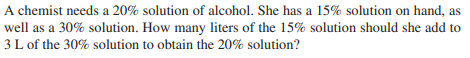### ¿Todavía tienes preguntas de matemáticas?

Pregunte a nuestros tutores expertos
Algebra
PreguntaA chemist needs a $$20 \%$$ solution of alcohol. She has a $$15 \%$$ solution on hand, as well as a $$30 \%$$ solution. How many liters of the $$15 \%$$ solution should she add to

$$3 L$$ of the $$30 \%$$ solution to obtain the $$20 \%$$ solution?